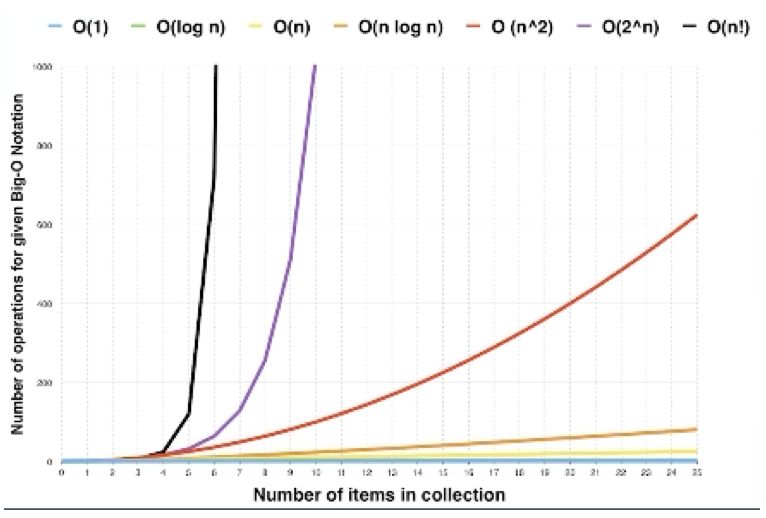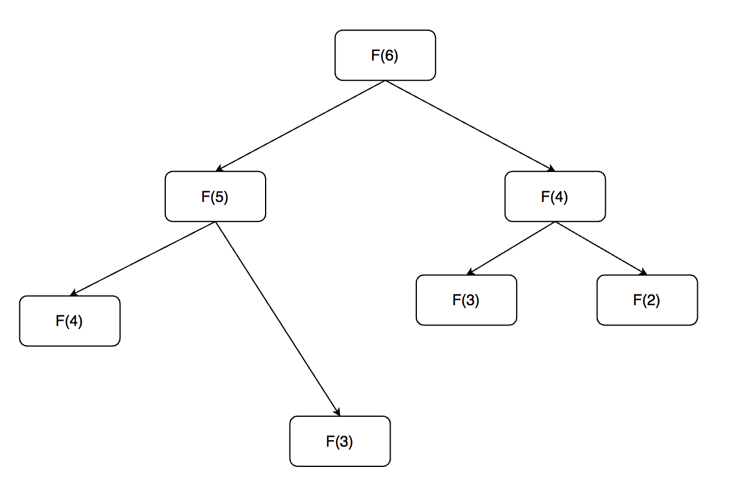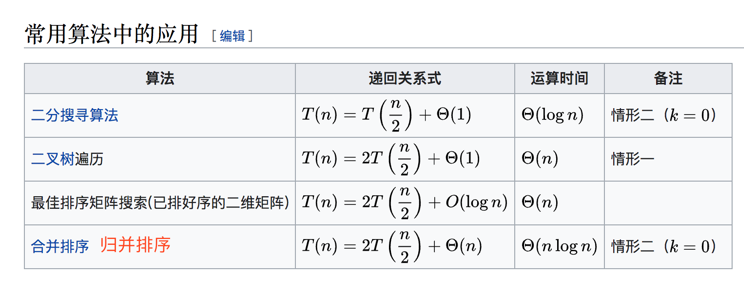# 一文吃透时间复杂度和空间复杂度﻿

## 时间复杂度

#### 最常见的时间复杂度有哪几种

• O(1)：Constant Complexity 常数复杂度

• O(log n)：Logarithmic ComPlexity 对数复杂度

• O(n)：Linear ComPlexity 线性时间复杂度

• O(n^2)：N square ComPlexity 平方

• O(n^3)：N cubic ComPlexity 立方

• O(2^n)：Exponential Growth 指数

• O(n!)：Factorial 阶乘

﻿

﻿

#### 如何来看一段代码的时间复杂度？

O(1)$n=100000;echo 'hello';﻿O(?)$n=100000;echo 'hello1';echo 'hello2';echo 'hello3';

﻿

O(n)for($i = 1;$i <= $n;$i++) {    echo 'hello';}﻿O(n^2)for($i = 1;$i <= $n;$i++) {    for($j = 1;$j <= $n;$j++) {        echo 'hello';    }}

﻿

﻿

O(log n)for($i = 1;$i <= $n;$i = $i*2) { echo 'hello';}﻿O(k^2)﻿fib($n) {    if ($n < 2) { return$n;    }        return fib($n-1) + fib($n-2);}

﻿﻿

﻿

﻿

从1加到2一直加到n，求它的和

$sum = 0;for ($i=1; $i <=$n; $i++) {$sum += $i;} ﻿ 方法二就是使用一个数学的求和公式： y = n*(n+1)/2 用这个公式，发现程序就只有一行了，所以它的时间复杂度就是O(1)了。所以可以看到，程序的不同方法，最后得到的结果虽然是一样的，但是它的时间复杂度很不一样 ﻿ #### 对于递归这种，如何分析时间复杂度？ 递归的话，关键就是要了解它的递归过程，总共执行了递归语句多少次。如果是循环的话，很好理解，n次的循环就执行了n次。那么递归的话，其实它是层层嵌套，其实很多时候，我们就是把递归它的执行顺序，画出一个树形结构，称之为它的递归状态的递归树。以前边的求Fibonacci(斐波拉契)数列的第n项为例 Fib：0,1,1,2,3,5,8,13,21...﻿F(n) = F(n-1)+F(n-2) 我之前面试的时候遇到过这么一道题，写的是最最简单的用递归的方式实现 fib($n) {    if($n < 2) { retuen$n;    }        return fib($n-1)+fib($n-2);}

﻿﻿

﻿

• 每多展开一层的话，运行的节点数就是上边一层的两倍，第一层只有1个节点，第二层有2个节点，第三层就有4个节点，再下一层就是8个节点。所以它每一层的话，它的节点数，也就是执行次数，是成指数增长的。由此可见，到n的时候，它就执行了2^n次

• 第二个可以观察到，有重复的节点出现在了执行的树里边，比如图中的F(4)和F(3)。如果将这个树继续展开，会发现F(4)、F(3)、F(2)都会被计算了很多次

﻿

﻿

#### 主定理

﻿

﻿﻿

﻿

﻿

﻿

﻿

﻿

﻿

﻿

﻿

﻿

﻿

﻿

﻿

﻿

## 空间复杂度

﻿

﻿

﻿﻿## 评论